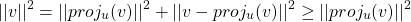## Projection methods in linear algebra numerics

Linear algebra classes often jump straight to the definition of a projector (as a matrix) when talking about orthogonal projections in linear spaces. As often as it happens, it is not clear how that definition arises. This is what is covered in this post.

## Orthogonal projection: how to build a projector

### Case 1 – 2D projection over (1,0)

It is quite straightforward to understand that orthogonal projection over (1,0) can be practically achieved by zeroing out the second component of any 2D vector, at last if the vector is expressed with respect to the canonical basis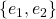. Albeit an idiotic statement, it is worth restating: the orthogonal projection of a 2D vector amounts to its first component alone.

How can this be put math-wise? Since we know that the dot product evaluates the similarity between two vectors, we can use that to extract the first component of a vector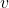. Once we have the magnitude of the first component, we only need to multiply that by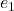itself, to know how much in the direction ofwe need to go. For example, starting from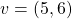, first we get the first component as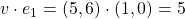; then we multiply this value by e_1 itself: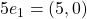. This is in fact the orthogonal projection of the original vector. Writing down the operations we did in sequence, with proper transposing, we get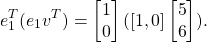One simple and yet useful fact is that when we project a vector, its norm must not increase. This should be intuitive: the projection process either takes information away from a vector (as in the case above), or rephrases what is already there. In any way, it certainly does not add any. We may rephrase our opening fact with the following proposition:

PROP 1: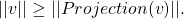This is can easily be seen through the pitagorean theorem (and in fact only holds for orthogonal projection, not oblique):